## conference papersJOURNAL OFAPPLIEDCRYSTALLOGRAPHY
ISSN: 1600-5767
Volume 40| Part s1| April 2007| Pages s373-s376

## Study of amorphous nanocrystalline thin silicon films by grazing-incidence small-angle X-ray scattering

aRudjer Boskovic Institute, 10000 Zagreb, Croatia, and bSincrotrone Trieste, SS 14 km 163.5, 34012 Basovizza (TS), Italy
*Correspondence e-mail: gracin@irb.hr

(Received 16 August 2006; accepted 15 January 2007; online 9 February 2007)

Thin Si films, with thicknesses between 100 and 400 nm, were deposited by radio frequency plasma enhanced chemical vapour deposition in silane gas (SiH4) highly diluted by hydrogen. The growing conditions were varied to obtain different degrees of crystal fractions and a variety in individual crystal sizes. The crystalline to amorphous volume fraction, as estimated by Raman spectroscopy, varied from 5 to 45% while the individual crystal sizes varied from 2 to 8 nm. The average density of the samples was estimated by using near infrared spectroscopy and the effective medium approximation. All samples were porous and contained void volume fraction between 15 and 25%. Grazing-incidence small-angle X-ray scattering has been performed at the ELETTRA synchrotron radiation source (Trieste, Italy). The scattering patterns of all examined samples indicate the presence of particles' in the bulk' of the thin films with gyration radii in the range of 2 to 5 nm. The higher values were found for the samples with a higher crystalline fraction. The size and the size distribution of particles' depend upon the deposition conditions. The samples which had been deposited with a higher discharge power and a lower silane fraction had larger particles and the roughness of their surface was higher.

### 1. Introduction

For a long time thin silicon films have been widely used in electronics and optoelectronics. A new era in this field started some 20 years ago with amorphous thin films, and it continued in the last decade with porous and nano-crystalline materials. By decreasing the crystal dimensions down to the nano – scale or by making amorphous structures, the optical, electrical and other properties of the material change. In that way, by combining the different degrees of structural ordering of the same material in single layer and/or multilayer structures, a great potential is obtained for many optoelectronic applications such as solar cells, flat screens, photo transistors, etc. (Green, 2000; Nozik, 2001). For a proper modelling of the materials growth, or for testing of the theoretical prediction of the quantum confinement of the electrons or the vibrational states, it is essential to establish a reliable diagnostic of the nanostructure. The advantages of using small-angle X-ray scattering (SAXS) in the diagnostic of the nano-structural properties of thin films, in comparison with transmission electron microscopy, is that SAXS provides information by averaging over a relatively large area, the sampling is easier and the method is non-destructive. In the case of thin film silicon, SAXS has been successfully applied to confirm the crucial role of nano-structure in light-induced degradation, in diffusion of hydrogen, in phase segregation, etc. (Prado et al., 1997; Williamson, 1995; Das et al., 2000; Shinar et al., 1999; Mahan et al., 2001; Gurman et al., 2000).

However, since grazing-incidence small-angle X-ray scattering (GISAXS) detects only the presence of objects which have an electron density that differs from the average electron density of the material (particles'), for a complete analysis this technique has to be combined with other methods. In this paper we study the nano-structural properties of thin films consisting of a mixture of amorphous and nano crystalline phases, deposited by the radio-frequency plasma-enhanced chemical vapour deposition (RF PECVD) method, by combining Raman spectroscopy and GISAXS.

### 2. Experimental

Thin silicon films were deposited by RF PECVD on a glass substrate in a capacitively coupled planar diode source. The working gas was silane diluted with hydrogen in the range 5–7% of silane in the gas mixture while the radio frequency (RF) plasma power densities were varied between 10 and 15 mW cm−2. On the top of all films, a thin amorphous silicon layer was deposited, under the same conditions for all the samples. In that way, it was possible to estimate the surface roughness through the presence or the absence of interference fringes.

Raman spectra were recorded by using a computerized DILOR Z24 triple monochromator with excitation by an argon ion laser, operating at 514.5 nm. The typical resolution was between 1 and 2 cm−1. The ratio of the areas under corresponding transversal optical (TO) phonon peaks of Raman spectra was taken as a measure of the crystal to amorphous fraction while the crystalline TO peak position, ωTO, was used for the estimation of the crystal size, dRaman, using the following formula (Jadkar et al., 2000):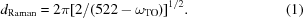In equation (1)ω represents the wavenumber (reciprocal of the wavelength) of the Raman shift, e.g. the difference between wavenumbers of excitation and emitted light, expressed in cm−1.

The spectral distribution of the refraction index was calculated from the transmittance and the reflectance measurements in the near infrared part of the spectra according to the Wemple DiDomenico model (Wemple & DiDomenico, 1971) and it was used for the estimation of the sample density by the effective medium approximation (Gracin et al., 2005). The typical density, estimated in this way, varied from sample to sample from 75 to 85% of the density of the crystalline samples, which assumes a substantial amount of voids in the samples.

GISAXS measurements were performed at the synchrotron ELETTRA, Trieste (Italy), at the SAXS beamline (Amenitsch et al., 1995), using an X-ray beam energy of 8 keV that corresponds to the wavelength, λ = 0.154 nm. The samples were mounted on a stepping-motor-controlled tilting stage with a step resolution of 0.010°. The grazing angle of incidence, αi, was selected in the range 0.4° < αi < 1.4°. X-ray scattering intensity spectra were acquired by a two-dimensional position sensitive charge-coupled device (CCD) detector, at a detector-to-sample distance L = 2 m. By changing the incident angle, it is possible to obtain the depth distribution of the particle' sizes. The GISAXS intensity is a convolution of scattering contributions from different depths attenuated according to the incoming and scattering angle. Therefore, an evaluation of the precise values would be very demanding and time consuming. However, for a rough estimation, the dominant contribution at the critical grazing incident angle comes from the near surface layer, while at the widest angles the contribution from the layer that is 300–400 nm below the surface becomes significant. The particle' sizes in the direction parallel to the surface and perpendicular to it were estimated by using the Guinier approximation for the analysis of the one-dimensional intensity distribution in two characteristic directions, parallel and perpendicular to the surface (Guinier & Fournet, 1955).

### 3. Results and discussion

The Raman spectra of a characteristic set of samples deposited by using different gas compositions are plotted in Fig. 1. The lowest spectrum corresponds to a sample deposited with the highest silane fraction and contain just a few percent of crystalline phase, which is seen as a small asymmetry in the TO phonon peak of the amorphous Si structure, close to 480 cm−1. The spectrum that is at the highest position in Fig. 1corresponds to a sample deposited with the lowest silane fraction and contain about 50% crystalline phase, which can be seen as a well distinguished contribution to the TO phonon peak close to 520 cm−1. An analogous effect has the increase of the RF power discharge in the range that was used here. By changing the deposition parameters in the whole range of power and silane dilution, it is possible to produce a high crystal fraction while maintaining the individual crystal sizes in the nanometre range, as can be seen in Fig. 2. The absence of a marked change in the individual crystal sizes suggests that the actual growing conditions favour more the nucleation rate than the crystal growth.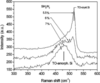Figure 1 Raman spectra of thin silicon samples deposited under different silane to hydrogen fractions.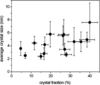Figure 2 The average individual crystal size as a function of the volume fraction of the crystalline phase.

The error bars in Fig. 2are relatively high since the width of the TO phonon peak is large which indicates a broad distribution of the individual particle sizes and inhomogeneity of the samples on the nanometre scale.

GISAXS patterns from samples deposited by the high and low plasma powers, measured at the critical angle and at 0.5° above it, are shown in Fig. 3. Here q = 4πsinθ/λ is the modulus of the scattering vector, where 2θ is the scattering angle and λ is the X-ray wavelength. The qz and qy are components in the direction perpendicular to the sample surface and parallel to the sample surface but perpendicular to the incoming beam, respectively.Figure 3 GISAXS of two 100 nm thick samples deposited under high, (a) and (b), and low, (c) and (d), discharge power, at the critical angle, (a) and (c), and 0.5° above it, (b) and (d); on both samples a top amorphous layer with 10 nm thickness was deposited.

The high power plasma sample (Figs. 3a and 3b) produces a round-shape scattering pattern that is typical for an irregular and relatively rough surface with the particles' that are either spherical or aspherical but randomly oriented. Since the Raman spectra results have shown that this sample has a relatively high nano-crystalline particle concentration, we suppose that the nanocrystals are also present at the sample surface in a high concentration. The pattern does not change significantly when the grazing angle is increased by 0.5°, which indicates the similar structure on the surface and within the film. This structure is most probably a consequence of the fact that the high power plasma is aggressive and its effect on the surface is similar to etching.

On the other hand the low power plasma seems less aggressive and therefore a more compact and smoother surface is produced. Also the correlation with the lower surface is maintained much better, which is evident from the fringes that are visible in GISAXS. In Fig. 3(c) the X-ray penetration is minimal (the grazing angle equals the critical angle) and the oscillation of the intensity is due to the 10 nm thick amorphous layer, deposited on the top of the film. Actually, these oscillations look more like a broadening of the scattering intensity in the horizontal direction at qz = 0.8 nm−1. However, when the grazing angle is increased, the X-ray penetration is much deeper and we can see fringes due to the film-to-substrate surface correlation, i.e. the scattering comes from the whole film of some 100 nm thickness.

In Fig. 4the average values for the radius of gyration of the particles' are shown, obtained by analysing the one-dimensional intensity distributions of GISAXS results in order to compare them with Raman results. Since Raman gives the average information from the volume defined by the illuminated area (1 mm × 10 mm) and the penetration depth of the laser beam (∼100 nm), the plotted points are the mean values of two angles, critical and 0.5° above, and two characteristic directions, parallel and perpendicular to the surface of the samples. The lines in Fig. 4are guide for the eyes denoting the range of expected values for RG. The scattering of data confirms the non uniformity of samples on the nanometre scale. The size of particles' slightly increases with crystal fraction but remains in the nanometre range, very similar to the case of the nano-crystals (Fig. 2). However, it is hard to establish a direct correlation since for the calculation of the real particle' sizes, their exact shape should be known and the contribution of the sample's porosity to the GISAXS has to be included.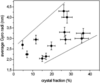Figure 4 Average radius of gyration as a function of the volume fraction of the crystalline phase.

The shape of the particles' changes as the crystalline fraction increases, as can be seen in Fig. 5(the plotted lines are only guides for the eyes). The error bars are large, which is consistent with the non-uniformity of the samples while the scattering of the data is most probably due to the fact that the nano-crystal formation is a result of several competing processes, such as the nucleation rate, the individual crystal growth and the plasma-surface etching. However, the results in Fig. 5represent the general trend of the changes in shape of the particles' with the increase in crystal fraction. For a low degree of crystallinity, the ratio between the radius of gyration parallel to the surface (RGH) and the radius of gyration perpendicular to the surface (RGV) is close to 1, i.e. the particles are spherical in shape, indicating a homogeneous nucleation. By increasing the crystal fraction, the sizes in the horizontal direction become dominant, suggesting the beginning of a formation of a columnar structure preferentially in the direction that is parallel to the substrate of the film.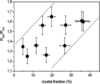Figure 5 The ratio between the average radius of gyration in a direction parallel to the sample surface (horizontal, RGH) and perpendicular to it (vertical, RGV) versus crystal fraction.

The weak correlation between crystal fraction and crystal size or shape of the particles' (Figs. 2, 4, 5) suggests that the details of growing conditions define these quantities. The influence of a variation in the discharge power on the depth distribution of the particles is illustrated in Fig. 6. The RGH and RGV are plotted for two samples deposited with different RF discharge power, as a function of the difference in GISAXS angles between critical and actual angle, Δαi, e.g. as a function of the scattering depth in the film. For lower power (10 mW cm−2, squares in Fig. 6) the gyro radii for the two characteristic directions are the same, indicating spherical particles and homogeneous growth, without intensive interference of plasma with the deposited film. For the sample deposited at higher power (15 mW cm−2, circles in Fig. 6), the gyro radii in the two directions are different, and the particles are larger for small Δαi (close to critical angle), i.e. close to the surface, which is characteristic for a columnar structure, most probably influenced by the plasma–surface interaction during the growth.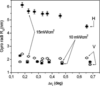Figure 6 Radius of gyration as a function of the difference of the actual GISAXS angle and the critical angle, Δαi, for two different powers of discharge used during the preparation, and in two directions, perpendicular (vertical, V) and parallel (horizontal, H) to the surface.

A similar effect on the depth distribution of the particles' shape has the gas composition, as can be seen in Fig. 7, where the ratio of the radius of gyration in a direction parallel to the surface (RGH) and perpendicular to it (RGV) is plotted as a function of the GISAXS angle for three gas compositions. The RGH/RGV ratio, i.e. the anisotropy in the shape of the particles, is the largest for the lowest silane fraction (full squares in Fig. 7) and has higher values when closer to surface. For slightly higher silane fraction, the anisotropy is lower and finally, for silane fraction of 7%, the particles are close to spherical shape (full circles in Fig. 7). The large variations of RGH/RGV ratio with incidence angle for these samples indicate an inhomogeneous structure across the depth.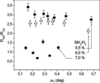Figure 7 The ratio between the average radius of gyration in a direction parallel to the surfaces of the samples (horizontal, RGH) and perpendicular to it (vertical, RGV) as a function of the difference of the actual GISAXS angle and the critical angle, Δαi.

The results plotted in Figs. 6and 7support the assumption that a lower silane fraction and a higher discharge power promote the formation of radicals in the plasma that thus intensively etch the Si film.

### 4. Conclusions

Thin silicon films, deposited by the RF PECVD method, using silane highly diluted with hydrogen, were examined using Raman spectroscopy and GISAXS. The Raman spectra showed that the samples contain up to 45% of nano-crystalline fraction, with individual crystal sizes ranging from 2 to 8 nm. The individual crystal sizes were larger for a higher crystalline fraction. The samples were porous, inhomogeneous on the nanometre scale and contained void volume fraction between 15 and 25%. The analysis of the GISAXS data indicated the presence of particles' in all deposited samples, with an average radius of gyration between 2 and 4.5 nm. With increasing crystalline fraction, the sizes of the particles' increased and their shape changed from near spherical towards elliptical indicating a preferential growth and a columnar structure. It was concluded from the study of the samples which were deposited under different RF power and different gas composition that a lower silane fraction and a higher discharge power promote the etching of the growing film.

### Acknowledgements

This work has been financially supported by the Croatian Ministry of Science, Education and Sport, Project No. TP-02/0098-35, and by the European Commission under contract No. INCO-CT-2004-509178.

### ReferencesJOURNAL OFAPPLIEDCRYSTALLOGRAPHY